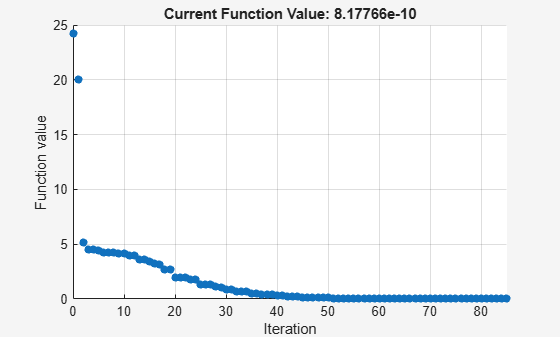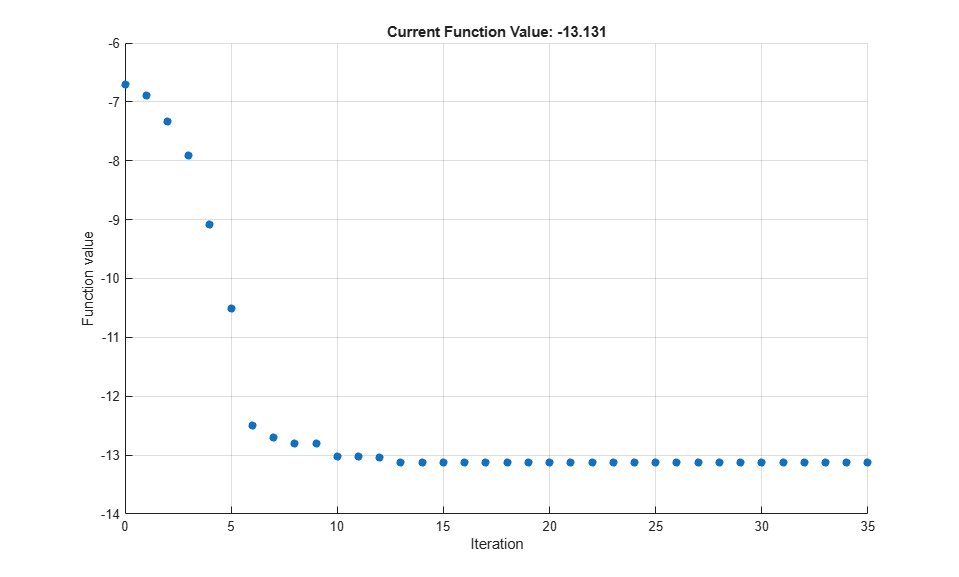# fminsearch

Find minimum of unconstrained multivariable function using derivative-free method

## Syntax

``x = fminsearch(fun,x0)``
``x = fminsearch(fun,x0,options)``
``x = fminsearch(problem)``
``````[x,fval] = fminsearch(___)``````
``````[x,fval,exitflag] = fminsearch(___)``````
``````[x,fval,exitflag,output] = fminsearch(___)``````

## Description

Nonlinear programming solver. Searches for the minimum of a problem specified by

`$\underset{x}{\mathrm{min}}f\left(x\right)$`

f(x) is a function that returns a scalar, and x is a vector or a matrix.

example

````x = fminsearch(fun,x0)` starts at the point `x0` and attempts to find a local minimum `x` of the function described in `fun`.```

example

````x = fminsearch(fun,x0,options)` minimizes with the optimization options specified in the structure `options`. Use `optimset` to set these options.```
````x = fminsearch(problem)` finds the minimum for `problem`, where `problem` is a structure.```

example

``````[x,fval] = fminsearch(___)```, for any previous input syntax, returns in `fval` the value of the objective function `fun` at the solution `x`.```
``````[x,fval,exitflag] = fminsearch(___)``` additionally returns a value `exitflag` that describes the exit condition.```

example

``````[x,fval,exitflag,output] = fminsearch(___)``` additionally returns a structure `output` with information about the optimization process.```

## Examples

collapse all

Minimize Rosenbrock's function, a notoriously difficult optimization problem for many algorithms:

`$f\left(x\right)=100\left({x}_{2}-{x}_{1}^{2}{\right)}^{2}+\left(1-{x}_{1}{\right)}^{2}.$`

The function is minimized at the point `x = [1,1]` with minimum value `0`.

Set the start point to `x0 = [-1.2,1]` and minimize Rosenbrock's function using `fminsearch`.

```fun = @(x)100*(x(2) - x(1)^2)^2 + (1 - x(1))^2; x0 = [-1.2,1]; x = fminsearch(fun,x0)```
```x = 1×2 1.0000 1.0000 ```

Set options to monitor the process as `fminsearch` attempts to locate a minimum.

Set options to plot the objective function at each iteration.

`options = optimset('PlotFcns',@optimplotfval);`

Set the objective function to Rosenbrock's function,

`$f\left(x\right)=100\left({x}_{2}-{x}_{1}^{2}{\right)}^{2}+\left(1-{x}_{1}{\right)}^{2}.$`

The function is minimized at the point `x = [1,1]` with minimum value `0`.

Set the start point to `x0 = [-1.2,1]` and minimize Rosenbrock's function using `fminsearch`.

```fun = @(x)100*(x(2) - x(1)^2)^2 + (1 - x(1))^2; x0 = [-1.2,1]; x = fminsearch(fun,x0,options)``````x = 1×2 1.0000 1.0000 ```

Minimize an objective function whose values are given by executing a file. A function file must accept a real vector `x` and return a real scalar that is the value of the objective function.

Copy the following code and include it as a file named `objectivefcn1.m` on your MATLAB® path.

```function f = objectivefcn1(x) f = 0; for k = -10:10 f = f + exp(-(x(1)-x(2))^2 - 2*x(1)^2)*cos(x(2))*sin(2*x(2)); end ```

Start at `x0 = [0.25,-0.25]` and search for a minimum of `objectivefcn`.

```x0 = [0.25,-0.25]; x = fminsearch(@objectivefcn1,x0) ```
```x = -0.1696 -0.5086 ```

Sometimes your objective function has extra parameters. These parameters are not variables to optimize, they are fixed values during the optimization. For example, suppose that you have a parameter `a` in the Rosenbrock-type function

`$f\left(x,a\right)=100\left({x}_{2}-{x}_{1}^{2}{\right)}^{2}+\left(a-{x}_{1}{\right)}^{2}.$`

This function has a minimum value of 0 at ${x}_{1}=a$, ${x}_{2}={a}^{2}$. If, for example, $a=3$, you can include the parameter in your objective function by creating an anonymous function.

Create the objective function with its extra parameters as extra arguments.

`f = @(x,a)100*(x(2) - x(1)^2)^2 + (a-x(1))^2;`

Put the parameter in your MATLAB® workspace.

`a = 3;`

Create an anonymous function of `x` alone that includes the workspace value of the parameter.

`fun = @(x)f(x,a);`

Solve the problem starting at `x0 = [-1,1.9]`.

```x0 = [-1,1.9]; x = fminsearch(fun,x0)```
```x = 1×2 3.0000 9.0000 ```

Find both the location and value of a minimum of an objective function using `fminsearch`.

Write an anonymous objective function for a three-variable problem.

```x0 = [1,2,3]; fun = @(x)-norm(x+x0)^2*exp(-norm(x-x0)^2 + sum(x));```

Find the minimum of `fun` starting at `x0`. Find the value of the minimum as well.

`[x,fval] = fminsearch(fun,x0)`
```x = 1×3 1.5359 2.5645 3.5932 ```
```fval = -5.9565e+04 ```

Inspect the results of an optimization, both while it is running and after it finishes.

Set options to provide iterative display, which gives information on the optimization as the solver runs. Also, set a plot function to show the objective function value as the solver runs.

```options = optimset('Display','iter','PlotFcns',@optimplotfval); ```

Set an objective function and start point.

```function f = objectivefcn1(x) f = 0; for k = -10:10 f = f + exp(-(x(1)-x(2))^2 - 2*x(1)^2)*cos(x(2))*sin(2*x(2)); end ```

Include the code for `objectivefcn1` as a file on your MATLAB® path.

```x0 = [0.25,-0.25]; fun = @objectivefcn1; ```

Obtain all solver outputs. Use these outputs to inspect the results after the solver finishes.

```[x,fval,exitflag,output] = fminsearch(fun,x0,options) ```
``` Iteration Func-count min f(x) Procedure 0 1 -6.70447 1 3 -6.89837 initial simplex 2 5 -7.34101 expand 3 7 -7.91894 expand 4 9 -9.07939 expand 5 11 -10.5047 expand 6 13 -12.4957 expand 7 15 -12.6957 reflect 8 17 -12.8052 contract outside 9 19 -12.8052 contract inside 10 21 -13.0189 expand 11 23 -13.0189 contract inside 12 25 -13.0374 reflect 13 27 -13.122 reflect 14 28 -13.122 reflect 15 29 -13.122 reflect 16 31 -13.122 contract outside 17 33 -13.1279 contract inside 18 35 -13.1279 contract inside 19 37 -13.1296 contract inside 20 39 -13.1301 contract inside 21 41 -13.1305 reflect 22 43 -13.1306 contract inside 23 45 -13.1309 contract inside 24 47 -13.1309 contract inside 25 49 -13.131 reflect 26 51 -13.131 contract inside 27 53 -13.131 contract inside 28 55 -13.131 contract inside 29 57 -13.131 contract outside 30 59 -13.131 contract inside 31 61 -13.131 contract inside 32 63 -13.131 contract inside 33 65 -13.131 contract outside 34 67 -13.131 contract inside 35 69 -13.131 contract inside Optimization terminated: the current x satisfies the termination criteria using OPTIONS.TolX of 1.000000e-04 and F(X) satisfies the convergence criteria using OPTIONS.TolFun of 1.000000e-04 x = -0.1696 -0.5086 fval = -13.1310 exitflag = 1 output = struct with fields: iterations: 35 funcCount: 69 algorithm: 'Nelder-Mead simplex direct search' message: 'Optimization terminated:...' ```The value of `exitflag` is `1`, meaning `fminsearch` likely converged to a local minimum.

The `output` structure shows the number of iterations. The iterative display and the plot show this information as well. The `output` structure also shows the number of function evaluations, which the iterative display shows, but the chosen plot function does not.

## Input Arguments

collapse all

Function to minimize, specified as a function handle or function name. `fun` is a function that accepts a vector or array `x` and returns a real scalar `f` (the objective function evaluated at `x`).

`fminsearch` passes `x` to your objective function in the shape of the `x0` argument. For example, if `x0` is a 5-by-3 array, then `fminsearch` passes `x` to `fun` as a 5-by-3 array.

Specify `fun` as a function handle for a file:

`x = fminsearch(@myfun,x0)`

where `myfun` is a MATLAB® function such as

```function f = myfun(x) f = ... % Compute function value at x```

You can also specify `fun` as a function handle for an anonymous function:

`x = fminsearch(@(x)norm(x)^2,x0);`

Example: `fun = @(x)-x*exp(-3*x)`

Data Types: `char` | `function_handle` | `string`

Initial point, specified as a real vector or real array. Solvers use the number of elements in, and size of, `x0` to determine the number and size of variables that `fun` accepts.

Example: `x0 = [1,2,3,4]`

Data Types: `double`

Optimization options, specified as a structure such as `optimset` returns. You can use `optimset` to set or change the values of these fields in the options structure. See Set Optimization Options for detailed information.

 `Display` Level of display (see Optimization Solver Iterative Display): `'notify'` (default) displays output only if the function does not converge.`'final'` displays just the final output.`'off'` or `'none'` displays no output.`'iter'` displays output at each iteration. `FunValCheck` Check whether objective function values are valid. `'on'` displays an error when the objective function returns a value that is `complex` or `NaN`. The default `'off'` displays no error. `MaxFunEvals` Maximum number of function evaluations allowed, a positive integer. The default is `200*numberOfVariables`. See Tolerances and Stopping Criteria. `MaxIter` Maximum number of iterations allowed, a positive integer. The default value is `200*numberOfVariables`. See Tolerances and Stopping Criteria. `OutputFcn` Specify one or more user-defined functions that an optimization function calls at each iteration, either as a function handle or as a cell array of function handles. The default is none (`[]`). See Optimization Solver Output Functions. `PlotFcns` Plots various measures of progress while the algorithm executes. Select from predefined plots or write your own. Pass a function handle or a cell array of function handles. The default is none (`[]`): `@optimplotx` plots the current point.`@optimplotfunccount` plots the function count.`@optimplotfval` plots the function value. For information on writing a custom plot function, see Optimization Solver Plot Functions. `TolFun` Termination tolerance on the function value, a positive scalar. The default is `1e-4`. See Tolerances and Stopping Criteria. Unlike other solvers, `fminsearch` stops when it satisfies both `TolFun` and `TolX`. `TolX` Termination tolerance on `x`, a positive scalar. The default value is `1e-4`. See Tolerances and Stopping Criteria. Unlike other solvers, `fminsearch` stops when it satisfies both `TolFun` and `TolX`.

Example: `options = optimset('Display','iter')`

Data Types: `struct`

Problem structure, specified as a structure with the following fields.

Field NameEntry

`objective`

Objective function

`x0`

Initial point for `x`

`solver`

`'fminsearch'`

`options`

Options structure such as returned by `optimset`

Data Types: `struct`

## Output Arguments

collapse all

Solution, returned as a real vector or real array. The size of `x` is the same as the size of `x0`. Typically, `x` is a local solution to the problem when `exitflag` is positive.

Objective function value at the solution, returned as a real number. Generally, `fval` = `fun(x)`.

Reason `fminsearch` stopped, returned as an integer.

 `1` The function converged to a solution `x`. `0` Number of iterations exceeded `options.MaxIter` or number of function evaluations exceeded `options.MaxFunEvals`. `-1` The algorithm was terminated by the output function.

Information about the optimization process, returned as a structure with fields:

 `iterations` Number of iterations `funcCount` Number of function evaluations `algorithm` `'Nelder-Mead simplex direct search'` `message` Exit message

## Tips

• `fminsearch` only minimizes over the real numbers, that is, the vector or array x must only consist of real numbers and f(x) must only return real numbers. When x has complex values, split x into real and imaginary parts.

• Use `fminsearch` to solve nondifferentiable problems or problems with discontinuities, particularly if no discontinuity occurs near the solution.

## Algorithms

`fminsearch` uses the simplex search method of Lagarias et al. . This is a direct search method that does not use numerical or analytic gradients as in `fminunc` (Optimization Toolbox). The algorithm is described in detail in fminsearch Algorithm. The algorithm is not guaranteed to converge to a local minimum.

## Alternative Functionality

### App

The Optimize Live Editor task provides a visual interface for `fminsearch`.

 Lagarias, J. C., J. A. Reeds, M. H. Wright, and P. E. Wright. “Convergence Properties of the Nelder-Mead Simplex Method in Low Dimensions.” SIAM Journal of Optimization. Vol. 9, Number 1, 1998, pp. 112–147.